Courses

# Mathematics Test 7 - 3-D Vector, Continuity

## 30 Questions MCQ Test JEE Main Mock Test Series 2020 & Previous Year Papers | Mathematics Test 7 - 3-D Vector, Continuity

Description
This mock test of Mathematics Test 7 - 3-D Vector, Continuity for JEE helps you for every JEE entrance exam. This contains 30 Multiple Choice Questions for JEE Mathematics Test 7 - 3-D Vector, Continuity (mcq) to study with solutions a complete question bank. The solved questions answers in this Mathematics Test 7 - 3-D Vector, Continuity quiz give you a good mix of easy questions and tough questions. JEE students definitely take this Mathematics Test 7 - 3-D Vector, Continuity exercise for a better result in the exam. You can find other Mathematics Test 7 - 3-D Vector, Continuity extra questions, long questions & short questions for JEE on EduRev as well by searching above.
QUESTION: 1

### Let p and q be the position vectors of P and Qrespectively, with respect to O and |p| =p, |q| = q.The points R and S divide PQ internally and externally in the ratio 2:3 respectively. If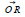and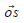are perpendicular, then

Solution: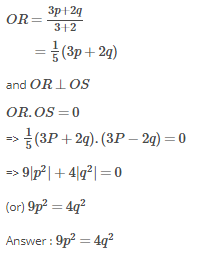QUESTION: 2

Solution:
QUESTION: 3

### The unit vectors perpendicular to vectors i–j and i+j forming a right handed system is

Solution:
QUESTION: 4
If A = 2i+2j+3k, B = –i+ 2j+ k and C = 3i+ j, then A +t B is perpendicular to C if t is equal to
Solution:
QUESTION: 5
If the vectors a,b and c form the side BC,CA and AB respectively, of a triangle ABC, then
Solution:
QUESTION: 6
Let the vectors a,b,c and d be such that (a×b)×(c×d) =0. Let P1 and P2 be planes determined by the pairs ofvectors a,b and c,d respectively. Then the angle between P1 and P2 is
Solution:
QUESTION: 7
If x and y are two unit vectors and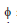is the angle between them, then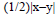is equal to
Solution:
QUESTION: 8

If a and b are two unit vectors such that a+2b and5a–4b are perpendicular to each other, then the angle between a and b is

Solution:
QUESTION: 9

Let a and b be two non-collinear unit vectors. if u = a–(a.b) b and v = a×b, then |v| is

Solution:
QUESTION: 10

If a.b and c are three non-coplanar vecotrs, then (a b c).[(a b)×(a c)] equals

Solution:
QUESTION: 11

If a, b and c are unit coplanar vectors, then the scalar triple product [2a–b 2b–c 2c–a] =

Solution:
QUESTION: 12
If a,b,c be three non-coplanar vectors, then i. [a+b,b+c,c+a] =, ii. [a–b,b–c,c–a] =
iii. [a×b,b×c,c×a] =
Solution:
QUESTION: 13
If a,b,c are non-coplanar unit vectors usch that a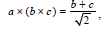(b and c non-coplanar) then the
angle between a and b is
Solution:
QUESTION: 14

If u = i×(a×i) j×(a×j) k×(a×b), then

Solution:
QUESTION: 15

Let a, b and c be three vectors with magnitudes 1, 1 and 2 respectively. If ax(axc) + b = 0, then the acute angle between a and c is?

Solution:
QUESTION: 16
The directional cosines of the line which is perpendicular to the lines with direction cosines
proportional to 6,4,–4 and –6,2,1 is
Solution:
QUESTION: 17
Angle between the lines whose direction cosine is
given by l m n=0=l2 m2–n2 is
Solution:
QUESTION: 18

The lines whose direction cosine are given by the relations a2l b2m c2n = 0 and mn nl lm = 0 are parallel if

Solution:
QUESTION: 19
The point of intersection of the line drawn form thevertices of any tetrahedran to the centroid of opposite faces divide the distance from each vertex to the opposite face in ratio
Solution:
QUESTION: 20
If vertices of tetrahedron are (1,2,3), (2,3,5),(3,–1,2) and (2,1,4) then its centroid is
Solution:
QUESTION: 21
If P,Q,A,B are (1,2,5),(–2,1,3),(4,4,2) and (2,1,–4) then the projection of PQ on AB is
Solution:
QUESTION: 22
Let the coordinates of A,B,C are (1,8,4),(0,–11,4), (2,–3,1) respectively. The coordinate of a point D
which is foot of the perpendicular from A on BC is
Solution:
QUESTION: 23
The coordinates of A,B,C are A (–1,2,–3), B(5,0,–6), C(0,4,–1). The direction cosines of the internal beisector of angle BAC are proportional to
Solution:
QUESTION: 24
Three line with direction ratios 1,1,2;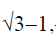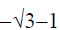,4  and,4 make
Solution:
QUESTION: 25
If acute angle between the planes 2x+ky+z = 6 and x+y+2z = 3 id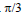,then k equals
Solution:
QUESTION: 26
Equation of the plane passing through the points (0,0,1),(1,0,1) and (1,–1,0) is
Solution:
QUESTION: 27

Equation of the plane through the point (4,5,1) and its normal is the line joning the points (3,4,2) and (1,1,1) is

Solution:
QUESTION: 28
If y =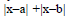then
Solution:
QUESTION: 29

Number of points where f(x) =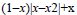is not differentiable is

Solution:
QUESTION: 30
The set of points where f(x)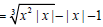is not differentiable is
Solution: